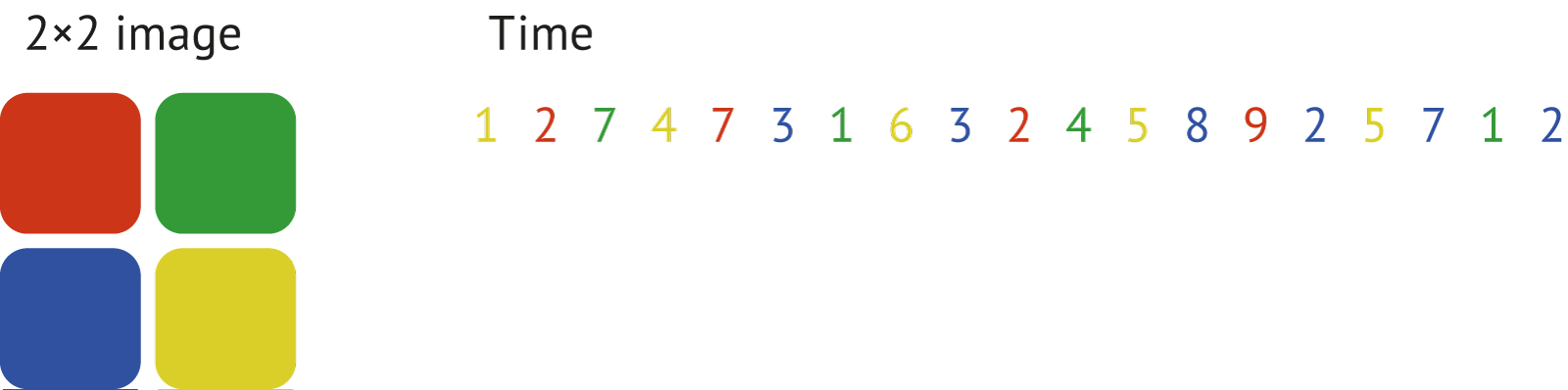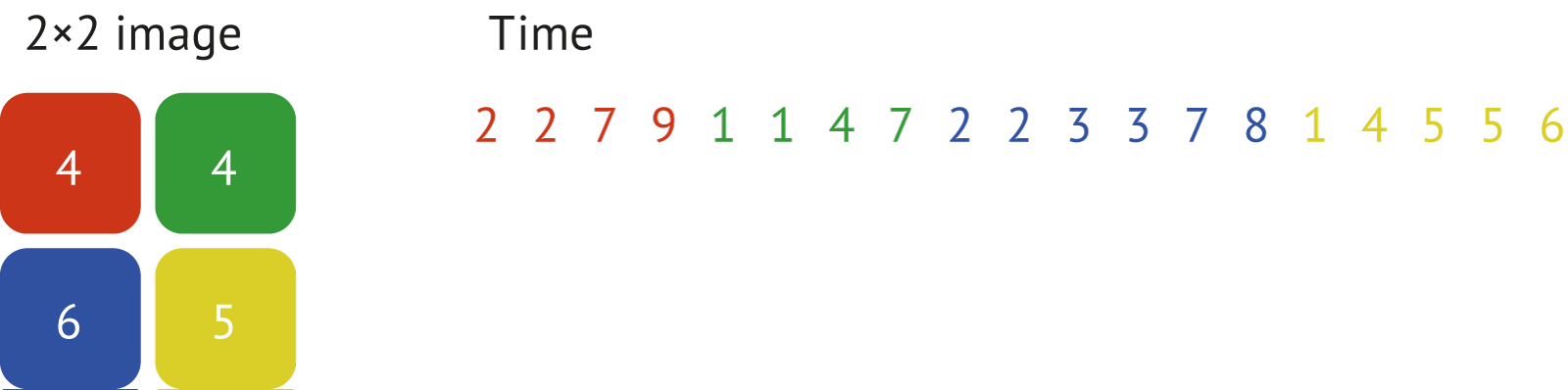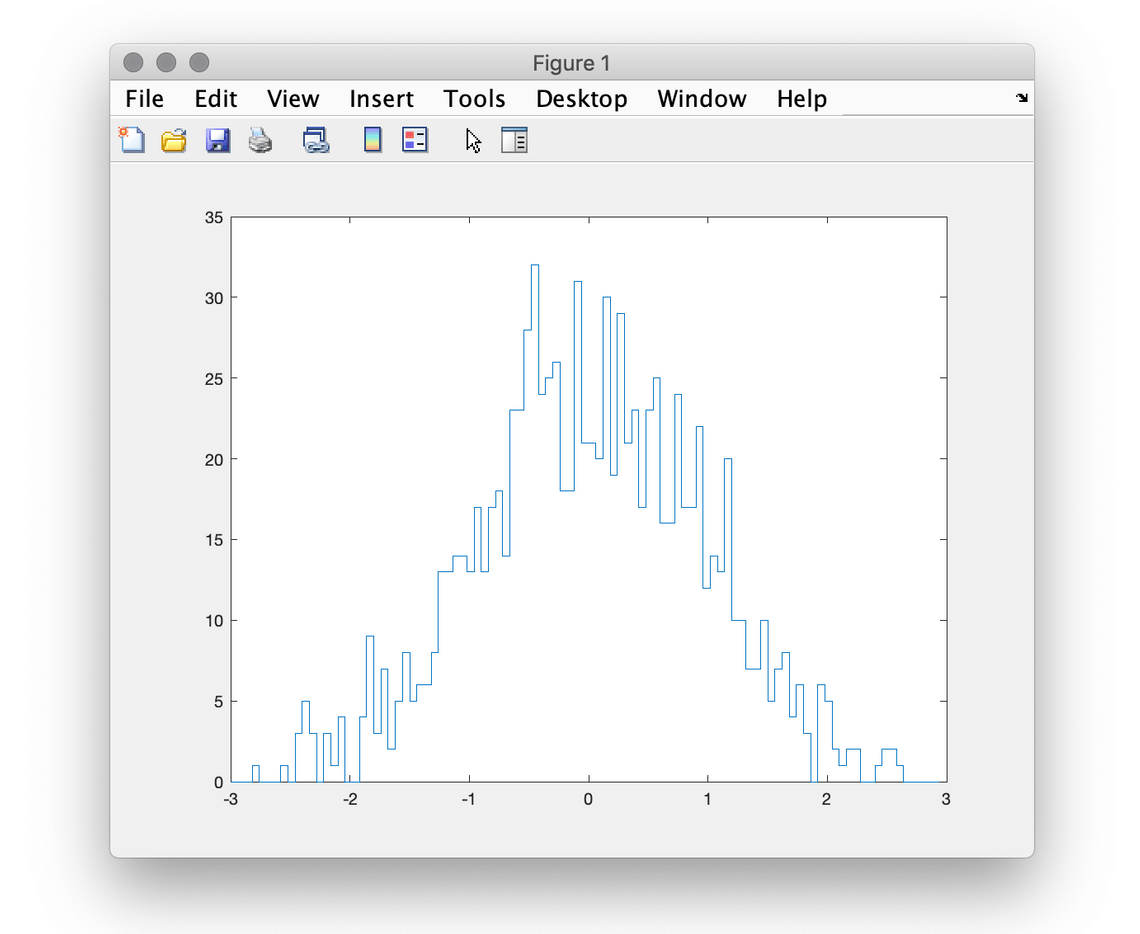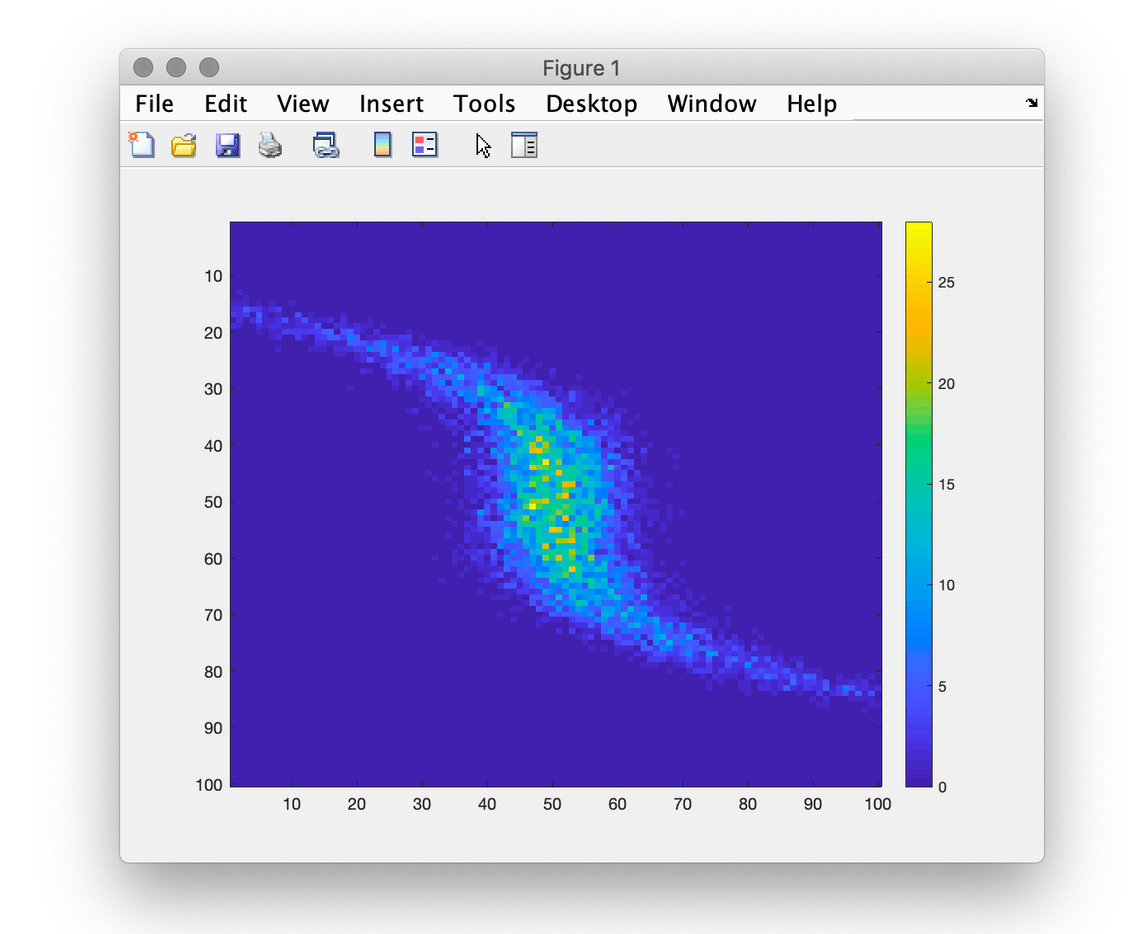### Photonscore Matlab Toolbox

MATLAB® computing environment has been our data analysis workign horse for many years we were working in research. As a company we are happy to share our well-tested toolbox for FLIM analysis with our customers.

complete example of using a toolbox to analyse MIET data set. Full source code and datafiles are available here (1.92 Gb).

##### Syntax
h = edges_hist_1d(x, edges)
h = edges_hist_1d(x, edges, w)
##### Description

edges_hist_1d builds one-dimensional histogram with bins defined by edges parameter of values x with corresponding weights w. When the w parameter is not given the weight of every value of x is assumed to be 1.

##### Parameters

x
Vector of numeric values to put into the histogram.

edges
Sorted vector on numeric values defining the edges of the bins.

w
Vector of numeric values of the weights of corresponding values.

##### Return Values

h
Vector of length length(edges)-1</span of numeric values of the histogram.

##### Examples

Build a histogram of the values sequence from 1 to 5 into two-bin histogram.

>> photonscore.edges_hist_1d([1 2 3 4 5], [0 3 10], [1 10 2 3 4])

ans =

11 9

Here the first bin results in 11 because only two values 1 and 2 fall into the interval [0; 3). These values have corresponding weights of 1 and 10 that in sum yields 11. The second bin is the sum of the weights 2, 3 and 4 corresponding to the values 3, 4 and 5. In this example unweighted (e.g. the weight of every value is 1) equivalent of x is:

>> x = [1 … % w = 1
2 2 2 2 2 2 2 2 2 2 … % w = 10
3 3 … % w = 2
4 4 4 … % w = 3
5 5 5 5] % w = 4

x =

Columns 1 through 11

1 2 2 2 2 2 2 2 2 2 2

Columns 12 through 20

3 3 4 4 4 5 5 5 5

>> photonscore.edges_hist_1d(x, [0 3 10])

ans =

11 9

##### Syntax
``info = photonscore.file_info(filename)``
##### Description
`file_info(filename)` retrieves metadata from the file given by filename.
##### Parameters
`filename` String specifying path to a .PHOTONS file.
##### Return values
`info` Structure holding file information with the following fields:
• `aat_frequencty`
Numeric value specifying frequency of absolute arrival time clock in Hz.
• `created`
String value formatted as a date and time of file eation.
• `detector_guid`
String holding globally unique LINCam system identifier.
• `dt_channel`
Numeric value of ΔT channel width in picoseconds.
• `dt_bias`
Numeric value of TAC bias as set by the user in acquisition program.
• `duration`
Numeric value of total duration of the recorded photon streams in seconds.
• `file_guid`
String holding globally unique file identifier.
• `photons_count`
Numeric 64 bit integer numeric value of total number of recorded photons.
• `raw_info_`
cell array of all metadata read from the file in raw form;
##### Examples
Get the information about gfp.photons file.
``````>> photonscore.file_info('gfp.photons')

ans =

struct with fields:

photons_count: 59905889
raw_info_: {26×3 cell}
created: '2019-02-28 14:31:23.166'
file_guid: '{d47b5c6b-bd16-401a-97ea-e63e58e872ee}'
dt_bias: 4095
dt_channel: 23.2900
aat_frequencty: 100000000
detector_guid: '{961a443c-697d-426b-b991-2abe8485695c}'
duration: 353.4260``````
The structure above reports there are about 59.9 million photons in this recording that lasted for 5 minutes and 53.426 seconds (353.4260).
##### Syntax
``````data = file_read(filename, dataset)
data = file_read(filename, dataset, offset, count)``````
##### Description
`data = file_read(filename, dataset)`
Retrieves all the data from the .PHOTONS of named dataset in the the file filename.
`data = file_read(filename, dataset, offset, count)`
Reads a subset of count long elements from dataset starting from the element pointed by offset.
##### Parameters
`filename`
String specifying the name of .PHOTONS file.
`dataset`
String specifying fully qualified dataset name in the .PHOTONS file given by filename parameter.
`offset`
Numeric value specifying the place to start reading the data. This is 0-based index. Prefer using uint64 type value to be able addressing the offsets in large datasets.
`count`
Numeric value specifying the amount of data to read.
`data`
##### Examples
Read an all `x` positions of the photons in the file `'gfp.photons'`:
``````>> photonscore.file_read('gfp.photons', '/photons/x')
ans =

59905889×1 uint16 column vector

2904
1519
1876
1803
1997
1858
1379
2111
3467
1867
....``````
Read five x positions starting from the forth value:
``````>> photonscore.file_read('gfp.photons', '/photons/x', 3, 5)

ans =

5×1 uint16 column vector

1803
1997
1858
1379
2111``````
Note that to start reading from the 4th element, e.g. `1803` offset parameter value 3 is given.
##### Syntax
``````rgb = photonscore.flim.iw_tau(i, l);
rgb = photonscore.flim.iw_tau(i, l, pal);
rgb = photonscore.flim.iw_tau(i, ri, l, rt, pal);``````
##### Description
`iw_tau(i, l)` computes `rgb` image of the intensity `i` weighted lifetime `l` using `'preview.png'` palette `iw_tau(i, l, pal)` computes `rgb` image of the intensity `i` weighted lifetime `l` using `pal` palette. `iw_tau(i, ri, l, rt, pal)` computes `rgb` image of the intensity weighted `i` lifetime `l` using `pal` palette cropping the dynamic range of intensity `i` into the range `ri` and limiting values of `l` into `rl` range. There are four palettes bundled with Photonscore toolbox:
##### Parameters
`i`
Numeric matrix of intensity values.
`ri`
Display range of intensity values. If this parameter is not provided the range would be generated to show the intensity from `0` counts up to a value that cut the to 10% off.
`i`
`li`
Display range of lifetime values. If this parameter is not provided the range would be generated to show the lifetimes cutting 5% of lower values and 5% of the higher values. In other words the range would be adjusted to show 90% of the whole range of lifetime values.
`pal`
Filename of the palette image file or numeric array of of size N×M×3. If the string is provided the function will try to read it with `imread` function in the working directory. Than the build-in palettes path will be used to find the file.
##### Syntax
``````[medi, mea] = photonscore.flim.medimean(fl)
[medi, mea] = photonscore.flim.medimean(fl, range)``````
##### Description
`medimean(fl, range)` loops through the sorted dataset `fl` computed by `flim.sort` function and returns median and mean lifetime images where `dt` falls inside `range`.
##### Parameters
`fl`
Sorted dataset structure.
`range`
A numeric two element vector of values constraining the range of time values.
##### Syntax
``````fli = photonscore.flim.sort(x, x_min, x_max, x_bins, y, dt)
fli = photonscore.flim.sort(x, x_min, x_max, x_bins, ...
y, y_min, y_max, y_bins, dt)``````
##### Description
`photonscore.flim.sort` builds a 2D histogram of the positions `x` and `y` in the half-closed intervals `[x_min, x_max)` and `[x_min, x_max)` with the number of bins defined by `x_bins` and `y_bins`. If the histogramming options for `y` are not provided those for `x` are used, e.g. `y_min = x_min``y_max = x_max` and `y_bins = x_bins`. The values of `dt` are ordered from smaller to larger. To illustrate the operation of the sorting function consider the dataset of 2×2 positional bins marked with the colors and corresponding `dt` values:After applying `photonscore.flim.sort` the resulting image (histogram) and the corresponding time values would look like shown here:The benefit of this data organization is an ability to extract very fast fluorescence decays for corresponding positions.
##### Parameters
`x, y`
Vectors of 16-bit unsigned integer values to form the intensity image.
`x_min, x_max, x_bins`
Numeric values defining the histogram interval for values `x`.
`y_min, y_may, y_bins`
Numeric values defining the histogram interval for values `y`.
`dt`
Vectors of of 16-bit unsigned integer values of the decay.
##### Return values
`fli` structure with the following fields:
• `image` `x_bins`×`y_bins` matrix of integer values holding number of counts of the bin.
• `time` is the vector of sorted numeric values of `dt` parameter.
##### Example
To reproduce the results depicted above one can use the following code fragment:
``````>> x  = uint16([2 1 2 2 1 1 2 2 1 1 2 2 1 1 1 2 1 2 1]);
>> y  = uint16([2 1 1 2 1 2 1 2 2 1 1 2 2 1 2 2 2 1 2]);
>> dt = uint16([1 2 7 4 7 3 1 6 3 2 4 5 8 9 2 5 7 1 2]);
>> fl = photonscore.flim.sort(x, 1, 3, 2, y, dt);``````
Evaluating the results would yield:
``````>> fl

fl =

struct with fields:

image: [2×2 int32]
time: [19×1 uint16]

>> fl.image'

ans =

2×2 int32 matrix

4   4
6   5

>> fl.time'

ans =

1×19 uint16 row vector

2   2   7   9   1   1   4   7   2   2   3   3   7   8   1   4   5   5   6``````
##### Syntax
``````h = hist_1d(x, x_min, x_max, x_bins)
h = hist_1d(x, x_min, x_max, x_bins, type)

h = hist_2d(x, x_min, x_max, x_bins, y)
h = hist_2d(x, x_min, x_max, x_bins, y, type)
h = hist_2d(x, x_min, x_max, x_bins,...
y, y_min, y_max, y_bins)
h = hist_2d(x, x_min, x_max, x_bins,...
y, y_min, y_max, y_bins, type)

h = hist_3d(x, x_min, x_max, x_bins, y, z)
h = hist_3d(x, x_min, x_max, x_bins, y, z, type)
h = hist_3d(x, x_min, x_max, x_bins,...
y,...
z, z_min, z_max, z_bins)
h = hist_3d(x, x_min, x_max, x_bins,...
y,...
z, z_min, z_max, z_bins, type)
h = hist_3d(x, x_min, x_max, x_bins,...
y, y_min, y_max, y_bins,...
z, z_min, z_max, z_bins)
h = hist_3d(x, x_min, x_max, x_bins,...
y, y_min, y_max, y_bins, ...
z, z_min, z_max, z_bins, type)``````
##### Description
`hist_1d` builds one-dimensional histogram of the values `x` in the half-closed interval `[x_min, x_max)` that is split into `x_bins` equally sized bins. `hist_2d` build two-dimensional histogram of the values `x` and `y` in half-closed intervals `[x_min, x_max)` for `x` and `[y_min, y_max)` for `y` split into `x_bins` and `y_bins` respectively. `hist_3d` build three-dimensional histogram of the values `x``y` and `z` in half-closed intervals `[x_min, x_max)` for `x``[y_min, y_max)` for `y` and `[z_min, z_max)` for `z` split into `x_bins``y_bins` and `z_bins` respectively.
##### Parameters
`x, y, z`
Vectors of numeric values of the same numeric type to form the histogram.
`x_min, x_max, x_bins`
Numeric values defining the histogram interval for values `x`.
`y_min, y_may, y_bins`
Numeric values defining the histogram interval for values `y`.
`z_min, z_maz, z_bins`
Numeric values defining the histogram interval for values `z`.
`type`
String value that defines the resulting histogram type. Supported types are: `uint8``uint16``uint32``int8`,`int16``int32``single` and `double`
##### Return values
Returns a histogram as a vector of numeric values.
##### Examples
In this example the function count the number of events falling into the intervals `[2, 3)` and `[3, 4]`. From the input vector only value `2` falls into the first bin and two values (`3` and `3.4`) are inside the second interval.
``````>> photonscore.hist_1d([1 2 3 3.4 5 10], 2, 4, 2)

ans =

1
2``````
Here we build a histogram of normally distributed values in a range `[-3, 3)` with `100` bins.
``````>> x = -3:0.06:2.94;
>> h = photonscore.hist_1d(randn(1000, 1), -3, 3, 100);
>> stairs(x, h)``````This example uses `10000` normally distributed values for `x` values and distorted for `y`.
``````>> x = randn(10000, 1);
>> y = randn(10000, 1)/3 + x.^3/3;
>> imagesc(photonscore.hist_2d(x, -3, 3, 100, y))
>> colorbar``````##### Syntax
``````data = read_photons(filename)
##### Description
`read_photon(filename)`
Reads all the photons attributes into the structure data.
`read_photon(filename, range_seconds)`
Reads a subset of photons data acquired within specified range.
##### Parameters
`filename`
String specifying the name of .PHOTONS file.
`range_seconds`
Two element vector of numeric values specifying the range of data in seconds.
##### Return values
`data` Structure holding the requested range of the data with the fields:
• `x`
Horisontal photon position
• `y`
Vertical photon position
• `dt`
Picosecond timing
##### Examples
Read the whole file into the memory.
``````>> photonscore.read_photons('gfp.photons')

ans =

struct with fields:

x: [59905824×1 uint16]
y: [59905824×1 uint16]
dt: [59905824×1 uint16]``````
Read the photons detected within 1.2 seconds starting from the second 3.
``````>> photonscore.read_photons('gfp.photons', [3 4.2])

ans =

struct with fields:

x: [203613×1 uint16]
y: [203613×1 uint16]
dt: [203613×1 uint16]``````